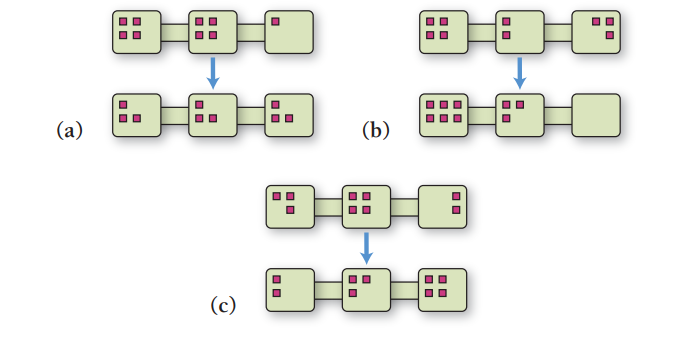×
Get Full Access to Chemistry: A Molecular Approach - 5 Edition - Chapter 19 - Problem 113
Get Full Access to Chemistry: A Molecular Approach - 5 Edition - Chapter 19 - Problem 113

×ISBN: 9780134874371 2047

## Solution for problem 113 Chapter 19

Chemistry: A Molecular Approach | 5th Edition

• Textbook Solutions
• 2901 Step-by-step solutions solved by professors and subject experts
• Get 24/7 help from StudySoup virtual teaching assistantsChemistry: A Molecular Approach | 5th Edition

4 5 1 362 Reviews
29
4
Problem 113

Consider the changes in the distribution of nine particles into three interconnected boxes shown here. Which has the most negative $$\Delta S$$ ?Text Transcription:

Delta S

Step-by-Step Solution:
Step 1 of 3

Quantum Numbers and Atomic Orbitals By solving the Schrödinger equation (Hψ = Eψ), we obtain a set of mathematical equations, called wave functions (ψ), which describe the probability of finding electrons at certain energy levels within an atom. A wave function for an electron in an atom is called an atomic orbital; this atomic orbital describes a region of space in which there is a high probability of finding the electron. Energy changes within an atom are the result of an electron changing from a wave pattern with one energy to a wave pattern with a different energy (usually accompanied by the absorption or emission of a photon of light). Each electron in an atom is described by four different quantum numbers. The first three (n, l, m)lspecify the particular orbital of interest, and the fourth (ms) specifies how many electrons can occupy that orbital. 1. Principal Quantum Number (n): n = 1, 2, 3, …, ∞ Specifies the energy of an electron and the size of the orbital (the distance from the nucleus of the peak in a radial probability distribution plot). All orbitals that have the same value of n are said to be in the same shell (level). For a hydrogen atom with n=1, the electron is in its ground state; if the electron is in the n=2 orbital, it is in an excited state. The total number of orbitals for a given n value is n2. 2. Angular Momentum (Secondary, Azimunthal) Quantum Number (l): l = 0, ..., n­1. Specifies the shape of an orbital with a particular prin

Step 2 of 3

Step 3 of 3

##### ISBN: 9780134874371

This full solution covers the following key subjects: . This expansive textbook survival guide covers 24 chapters, and 162 solutions. Since the solution to 113 from 19 chapter was answered, more than 201 students have viewed the full step-by-step answer. Chemistry: A Molecular Approach was written by Aimee Notetaker and is associated to the ISBN: 9780134874371. This textbook survival guide was created for the textbook: Chemistry: A Molecular Approach, edition: 5. The full step-by-step solution to problem: 113 from chapter: 19 was answered by Aimee Notetaker, our top Chemistry solution expert on 06/03/22, 04:20PM. The answer to “?Consider the changes in the distribution of nine particles into three interconnected boxes shown here. Which has the most negative $$\Delta S$$ ? Text Transcription:Delta S” is broken down into a number of easy to follow steps, and 26 words.

## Discover and learn what students are asking

Calculus: Early Transcendental Functions : Partial Derivatives
?In Exercises 7 - 38, find both first partial derivatives. $$z=y e^{y / x}$$

Unlock Textbook Solution